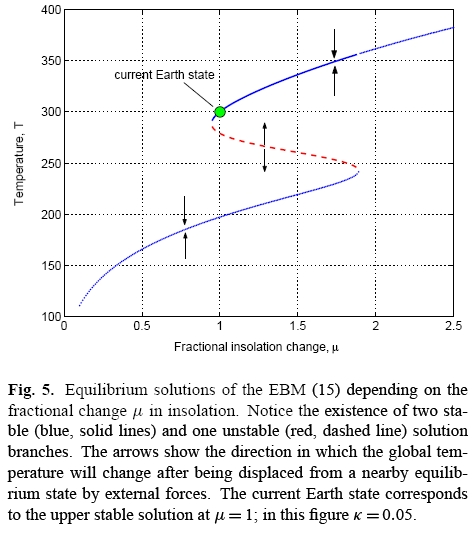# The Azimuth Project Another look at climate sensitivity (Rev #6, changes)

Showing changes from revision #5 to #6: Added | Removed | Changed

Note: Under construction.

$d T = \frac{\partial T}{\partial R} d R + \frac{\partial T}{\partial \alpha} d\alpha + \frac{\partial T}{\partial t} d t.$

## Azimuth Quote

The following paper describes a simple energy balance model with ice albedo feedback?, which exhibits a Snowball Earth phase:

Equations (11) and (12) in this paper have, for certain amounts of solar radiation falling on the Earth, three stationary solutions that correspond to possible climates for the Earth:Here ‘insolation’ is jargon for the amount of solar radiation reaching the Earth, and ‘EBM’ is an acronym for energy balance model. The dot shows the Earth’s climate now, in this simplified model. If the insolation were lowered there would be a tipping point, and the only solution would be a much cooler Snowball Earth. At somewhat higher insolations we see three phases: a stable warm phase, a stable Snowball Earth phase, and an unstable phase with an intermediate temperature, drawn as a dashed red line. At even higher insolations only the warm phase exists.

This situation is an example of a ‘fold catastrophe’.

When plotted as a function of ice line latitude (boundary of the polar ice caps) vs. insolation, instead of temperature vs. insolation, the equilibrium curve in the above figure is an example of the slope-stability theorem. This theorem states that the stability of the equilibrium solution depicted by such a curve is determined by its slope, with positive slopes corresponding to stable solutions, and negative slopes corresponding to unstable solutions.import numpy
import scipy
import matplotlib
from pylab import *

sigma = 5.6697e-8; Q0 = 342.5; kappa= 0.05;

def alpha(kappa,T):
c1 = 0.15; c2 = 0.7; Tc = 273.0;
return c1 + .5*c2*(1 - tanh(kappa*(T - Tc)))

def g(T):
m = 0.4; T0 = (1.9e-15)**(-1/6.);
return 1 - m*tanh((T/T0)**6)

def Ro(sigma,T):
return sigma*g(T)*T**4

def Ri(mu,Q0,kappa,T):
return mu*Q0*(1 - alpha(kappa,T))

def mu_eq(sigma,Q0,kappa,T):
return Ro(sigma,T)/Ri(1.,Q0,kappa,T)

def get_mu(mu0,a,omega,t):
mu = 1.*t;
mu[t < 0] = mu0;
mu[t >= 0] = mu0+a*sin(omega*t[t >=0])
return mu

T_init = 300.;
mu_init = mu_eq(sigma,Q0,kappa,T_init);

a = .5; omega = .1; c = 1.;

nt = 100.;
dt = 2*pi/(nt*omega);
nmin = -nt;
nmax = 9*nt;
time = arange(nmin, nmax, dt);

mu = get_mu(mu_init,a,omega,time);

Rnet = 1.*time;
T = 1.*time;

i = 0;
T_prev = 1.*T_init;
for t in time:
Rnet[i] = Ri(mu[i],Q0,kappa,T_prev)-Ro(sigma,T_prev)
T[i] = T_prev+(dt/c)*Rnet[i]
T_prev = T[i]
i = i+1;

subplot(311)
plot(time, mu,'b-', lw=2)
grid(True)
xlabel('Time')
ylabel('mu')

subplot(312)
plot(time, T,'b-', lw=2)
grid(True)
xlabel('Time')
ylabel('Temperature')

subplot(313)
Tp = arange(100.0, 400.0+1.0, 1.0)
plot(Tp, mu_eq(sigma,Q0,kappa,Tp), 'b-', T, mu, 'r-', lw=2)
grid(True)
xlabel('Temperature')
ylabel('mu')

show()